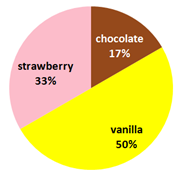# Charts

GR1

GR2

GR3

GR4

GR5

GR6

GR22

Pictographs

GR18

Dot Plot A simple graph that represents a set of data by using dots over a number line. Each X (or dot) is one result.Summarizing data using dot plots:

GR26

GR27

GR28

Line Graph  A graph used to show change and direction of change over a period of time.Summarizing Quantitative Data (Scatter Plots)

GR7

GR8

GR9

GR10

GR11

GR12

GR13

GR14

GR15

GR16

GR17

###### Measure and Representation of Data – Histogram

A special kind of bar graph that displays the frequency of data that has been organized into equal number groupings.  The number groupings cover all possible values of data, therefore there are no spaces between the bars.Histograms

GR19

GR20

###### Measure and Representation of Data – Circle Graph

A graph used to compare parts of a whole. The circle represents the whole and is separated into parts of the whole.Reading and Interpreting Pie or Circle Graphs:

GR21

GR23

GR24

Select a Graph

GR25

### Measure and Representation of Data  – Venn Diagram

Venn diagrams  are diagrams that show all hypothetically possible logical relations between a finite collection of sets. Draw a Venn diagram that shows the following sample space and events:

• All the integers from 1 to 12
• Even numbers
• Multiples of 3

The Venn diagram is as follows:Creating, Reading and Interpreting Venn Diagrams:

GR29

GR30

GR31

GR32

GR33

###### Stem-Leaf Plot

A Stem and Leaf Plot is a type of graph that is similar to a histogram but shows individual values.GR34

GR35

GR36

GR37

Measure and Representation of Data

To get a sense of the data’s distribution we use the five statistical summaries:

the (1) minimum, (2) maximum, (3) median (or second quartile), (4) the first quartile, and (5) the third quartile.

Example, Test scores:      60,70,70,70,85,85,85,90,100Population size: 9

Median: 85

Minimum: 60

Maximum: 100

First quartile: 70

Third quartile: 87.5

Interquartile Range: 17.5

We can use a steam-and-leaf plot to find and display the median, the Q1 and the Q3The median is at 85 and is marked by a circle. The Q1 is (70+70)/2=70  while the Q3 is (85+90)/2=87.5

### PR08      Range

###### Box and Whisker PlotGR38

GR39

GR40

GR41

Predict & Display Data Probability – Sample Space

Probability Help,

Probability 2 Help

Dependent Events Help,

Tree Diagram Help

ProbabilityVoc

Probability – Counting Principle Probability – Independent Event Probability Application